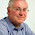# Artificial Intelligence Questions and Answers Set 4

## Artificial Intelligence

### Questions 31 to 40

31.
What is a Cybernetics?
 (a) Study of communication between two machines (b) Study of communication between human and machine (c) Study of communication between two humans (d) Study  of Boolean values (e) Study of communication between logic circuits.
32.
What is the goal of artificial intelligence?
 (a) To solve real-world problems (b) To solve artificial problems (c) To explain various sorts of intelligence (d) To extract scientific causes (e) To restrict problems.
33.
An algorithm is complete if
 (a) It terminates with a solution when one exists (b) It starts with a solution (c) It does not terminate with a solution (d) It has a loop (e) It has a decision parameter.
34.
Which is true regarding BFS?
 (a) BFS will get trapped exploring a single path (b) The entire tree so far been generated must be stored in BFS (c) BFS is not guaranteed to find a solution, if exists (d) BFS is nothing but Binary First Search (e) BFS is one type of sorting.
35.
What is a heuristic function?
 (a) A function to solve mathematical problems (b) A function which takes parameters of type string and returns an integer value (c) A function whose return type is nothing (d) A function which returns an object (e) A function that maps from problem state descriptions to measures of desirability.
36.
The traveling salesman problem involves n cities with paths connecting the cities. The time taken for traversing through all the cities, without knowing in advance the length of a minimum tour, is
 (a) O(n) (b) O(n2) (c) O(n!) (d) O(n/2) (e) O(2n).
37.
The problem space of means-end analysis has
 (a) An initial state and one or more goal states (b) One or more initial states and one goal state (c) One or more initial states and one or more goal state (d) One initial state and one goal state (e) No goal state.
38.
An algorithm A is admissible if
 (a) It is not guaranteed to return an optimal solution when one exists (b) It is guaranteed to return an optimal solution when one exists (c) It returns more solutions, but not an optimal one (d) It guarantees to return more optimal solutions (e) It returns no solutions at all.
39.
Knowledge may be
I.     Declarative.
II.     Procedural.
III.    Non-procedural.
 (a) Only (I) above (b) Only (II) above (c) Only (III) above (d) Both (I) and (II) above (e) Both (II) and (III) above.
40.
Idempotency law is
I.     P Ú P = P.
II.     P Ù P = P.
III.    P + P = P.
 (a) Only (I) above (b) Only (II) above (c) Only (III) above (d) Both (I) and (II) above (e) Both (II) and (III) above.

#### Answers

 31 Answer : (b) Reason  :       Cybernetics is Study of communication between human and machine 32 Answer : (c) Reason  :       The scientific goal of artificial intelligence is to explain various sorts of intelligence 33 Answer : (a) Reason  :       An Algorithm is complete if It terminates with a solution when one exists. 34 Answer : (b) Reason  :       Regarding BFS-The entire tree so far been generated must be stored in BFS. 35 Answer : (e) Reason  :       Heuristic function is a function that maps from problem state descriptions to measures of desirability 36 Answer : (c) Reason  :       The traveling salesman problem involves n cities with paths connecting the cities. The time       taken for traversing through all the cities, without knowing in advance the length of a minimum tour, is O(n!) 37 Answer : (a) Reason  :       The problem space of means-end analysis has an initial state and one or more goal states 38 Answer : (b) Reason  :       An algorithm A is admissible if It is guaranteed to return an optimal solution when one exists. 39 Answer : (d) Reason  :       Knowledge may be declarative and  procedural 40 Answer : (a) Reason  :       Idempotency Law is P V P = P

<< Prev  1 ...  2 ...  3 ...  4 ...  5 ...  6 ...  Next  >>

#### 1 comment :

1.Today's AI interaction was a spam chat bot. Spam is an excellent example of big numbers times times tiny probabilities.best virtual assistant